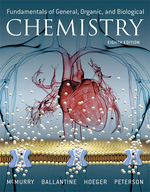×
Get Full Access to Fundamentals Of General, Organic, And Biological Chemistry (Mastering Chemistry) - 8 Edition - Chapter 9 - Problem 9.72
Get Full Access to Fundamentals Of General, Organic, And Biological Chemistry (Mastering Chemistry) - 8 Edition - Chapter 9 - Problem 9.72

×ISBN: 9780134015187 2044

## Solution for problem 9.72 Chapter 9

Fundamentals of General, Organic, and Biological Chemistry (Mastering Chemistry) | 8th Edition

• Textbook Solutions
• 2901 Step-by-step solutions solved by professors and subject experts
• Get 24/7 help from StudySoup virtual teaching assistantsFundamentals of General, Organic, and Biological Chemistry (Mastering Chemistry) | 8th Edition

4 5 1 309 Reviews
28
1
Problem 9.72

Calculate the mass needed for each of the following ion equivalents:

(a) 0.25 Eq $$\mathrm{Ca}^{2+}$$

(b) 75 mEq $$\mathbf{K}^{+}$$

(c) 199 mEg $$\mathrm{SO}_{4}^{2-}$$

(d) 0.65 Eq $$\mathrm{PO}_{4}^{3-}$$

Text Transcription:

Ca62+

K^+

SO_4^2-

PO_4^3-

Step-by-Step Solution:
Step 1 of 3

18.1-18.3 Wednesday, April 6, 2018:05 AM Lectures Page 1 Lectures Page 2 Lectures Page 3 Lectures Page 4 Lectures Page 5 Lectures Page 6 Lectures Page 7 Lectures Page 8 18.4 Friday, April 8, 208:14 AM Lectures Page 1 Lectures Page 2 Lectures Page 3 17.8-17.9 Monday, April 4, 20163:09 PM Lectures Page 1 Lectures Page 2 Lectures Page 3 Lectures Page 4 Lectures Page 5 Lectures Page 6

Step 2 of 3

Step 3 of 3

##### ISBN: 9780134015187

The answer to “?Calculate the mass needed for each of the following ion equivalents: (a) 0.25 Eq $$\mathrm{Ca}^{2+}$$ (b) 75 mEq $$\mathbf{K}^{+}$$(c) 199 mEg $$\mathrm{SO}_{4}^{2-}$$(d) 0.65 Eq $$\mathrm{PO}_{4}^{3-}$$Text Transcription:Ca62+K^+SO_4^2-PO_4^3-” is broken down into a number of easy to follow steps, and 26 words. Since the solution to 9.72 from 9 chapter was answered, more than 200 students have viewed the full step-by-step answer. This textbook survival guide was created for the textbook: Fundamentals of General, Organic, and Biological Chemistry (Mastering Chemistry), edition: 8. This full solution covers the following key subjects: . This expansive textbook survival guide covers 281 chapters, and 456 solutions. The full step-by-step solution to problem: 9.72 from chapter: 9 was answered by Aimee Notetaker, our top Chemistry solution expert on 04/25/22, 03:57PM. Fundamentals of General, Organic, and Biological Chemistry (Mastering Chemistry) was written by Aimee Notetaker and is associated to the ISBN: 9780134015187.

## Discover and learn what students are asking

Calculus: Early Transcendental Functions : Inverse Trigonometric Functions: Integration
?In Exercises 1-20, find the indefinite integral. $$\int \frac{1}{x \sqrt{x^{4}-4}} d x$$

Chemistry: The Central Science : The Chemistry of Life: Organic and Biological Chemistry
?Identify the carbon atom(s) in the structure shown that has (have) each of the following hybridizations: (a) s p^{3}, (b) sp,

Unlock Textbook Solution